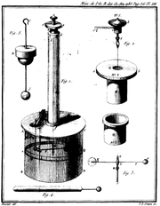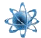xElectric charge
Posts  1 - 1  of  110126048
A thin rod of length 2L lies along the x axis with its center at the origin. The rod has a linear charge density given by p(x)=p0(x/L)^2 where x is the distance from the origin and p0 is positive. The electric potential at a point on the x axis at x=2L is what?